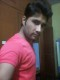# Queue Implementation in Java

A queue is a linear data structure which follows the FIFO (first-in first-out) principle. That means the object which is inserted first will be the first one out, followed by the object which was inserted next.

The queue supports below core operations –

1. `enqueue:` Inserts an item at the rear of the queue.

2. `dequeue:` Removes the object from the front of the queue thereby decremeting queue size by one.

3. `peek:` Returns the object at the front of the queue without removing it.

4. `isEmpty:` Tests if the queue is empty or not.

5. `size:` Returns the number of elements present in the queue.

Below is the Queue implementation in Java using an array.

## Java

Output:

Inserting 1
Inserting 2
Inserting 3
Front element is: 1
Removing 1
Front element is: 2
Queue size is 2
Removing 2
Removing 3
Queue Is Empty

The time complexity of `enqueue()`, `dequeue()`, `peek()`, `isEmpty()` and `size()` functions is constant. i.e. O(1).

Java’s library also contains a Queue interface that specifies queue operations. Below is an example of Queue interface using LinkedList implementation.

## Java

Output:

Front element is: A
Front element is: C
Queue size is 2
Queue is not Empty(1 votes, average: 5.00 out of 5)Loading...

Please use our online compiler to post code in comments. To contribute, get in touch with us.
Like us? Please spread the word and help us grow. Happy coding 🙂